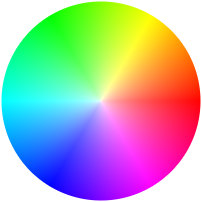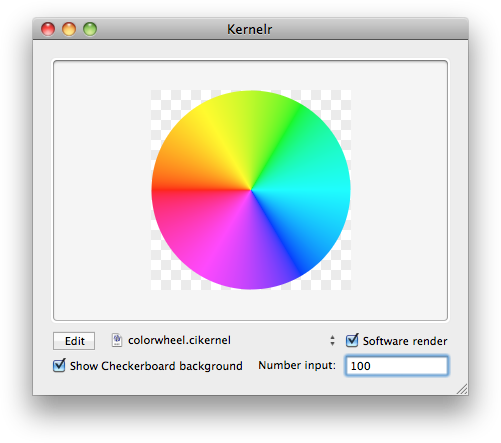The Shape of Everything
 Making a Color Wheel in Core Image 2010.07.29 01:07 PM For some reason recently, I wanted to see if I could write a Core Image filter to make a color wheel. Ok, that's kind of a lie. I recently came across Apple's private CIColorWheelGenerator filter and I was really intrigued at how they did it. Here's what the output from it looks like:(Exhibit A, the output from Apple's private color wheel generator) I didn't spend much time on it, but my friend George Codillo did, and here's what he came up with (along with some help from Andy Finnell's HSB mixer CI Kernel).(Exhibit B, the color wheel in Acorn's Kernelr sample code, as coded up by George.) And here's his code: ```bool isOdd(int v) { float dividend = float(v) / 2.0; return dividend != floor(dividend); } vec4 hsvToRgb(vec4 hsv) { float h = hsv.r; float s = hsv.g; float v = hsv.b; int i = int(floor(h)); float f = isOdd(i) ? h - float(i) : 1.0 - (h - float(i)); float m = v * (1.0 - s); float n = v * (1.0 - s * f); vec4 result = (i == 0) ? vec4(v, n, m, hsv.a) : ((i == 1) ? vec4(n, v, m, hsv.a) : ((i == 2) ? vec4(m, v, n, hsv.a) : ((i == 3) ? vec4(m, n, v, hsv.a) : ((i == 4) ? vec4(n, m, v, hsv.a) : ((i == 5) ? vec4(v, m, n, hsv.a) : vec4(v, n, m, hsv.a)))))); return (h == -1.0) ? vec4(v, v, v, hsv.a) : result; } kernel vec4 colorWheel(sampler src, float radius) { vec2 center = vec2(radius, radius); vec2 v0 = destCoord(); vec2 v1 = vec2(v0.x, radius); float a = distance(v0, v1); float b = distance(v1, center); float angle = atan(a,b); float pi = 3.14159265; angle = (v0.x>radius)?((v0.y>radius)? (atan(b,a)+pi/2.0):atan(a,b)+pi): (v0.y